Algebra

Equations

Inequalities

Graphs

Numbers

Calculus

Matrices

Tutorials

# Linear Equation in One Variable

The following are examples of statements of equality of two algebraic expressions

1. 4 (x-3)=4 x-12
2. x2+3x = x3+3x
3. x+2=10
4. x2-3 x = 18
5. x+5 = x-7
6. x2-4 x = x2-4 (x+3)

Statements 1 and 2 are true for all permissible values of 4. Such statements are called identities. Note that it is not permissible to assign the value 0 to x in statement 2.

Statements 3 and 4 are true for some but not all values of x. Statement 3 is true only if is 8. Statement 4 is true only if x is 3 or 6. Such statements are called equations.

Statements 5 and 6 are not true for any value of x and are called false statements.

DEFINITION The set of all numbers that satisfy an equation is called the solution set of the equation. The elements in the solution set are called the roots of the equation

To check whether a value of the variable is a root of the equation, substitute the value for the variable in the equation to see if the value of the right side of the equation is equal to the value of the left side of the equation.

DEFINITION        An equation is said to be linear if the variables in the equation all have exponents of 1 and if no term of the equation has more than one variable as a factor.

The equation x+y-z = 1 is a linear equation in x,y, and z.

The equation x2+x-6 is not a linear equation.

The equation 1 x+xy = 9 is not a linear equation in x and y.

This chapter deals with linear equations in one variable

Equivalent equations

DEFINITION Two equations are said to be equivalent if they have the same solution set.

The equations 5 x+7 = 2 and x = 1 are equivalent. The two equations have the same solution set, { 1}.

The solution sets of some equations are obvious by inspection. The solution set of the equation x+4= 10 is {6}, since 6 is the only number that, when added to 4, equals 10. The solution set of the equation 5 x-2 = 3 (x+4) is not so obvious.

To solve an equation, that is, to ﬁnd its solution set, two theorems can be applied to get an equivalent equation whose solution is obvious.

THEOREM 1 lf P,Q, and T are polynomials in the same variable and P = Q is an equation, then P-Q and P+T = Q+T are equivalent.

Theorem 1 states that, given an equation P = Q, we can add any polynomial T in the same variable as P and Q to both sides of the equation, thus obtaining an equivalent equation P+T = Q+T.

The two equations 4 x-1 = 3 x+5 and 4 x-1+(1-3 x) = 3 x+5+(1-3 x), which simpliﬁes to x = 6, are equivalent. Their solution set is {6}.

THEOREM 2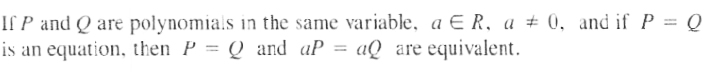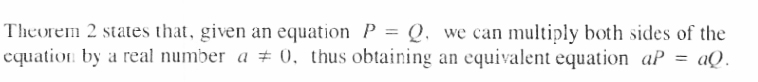The two equations x = 2 and 5 (x) = 5 (2), that is, 5 x = 10, are equivalent. Their solution set is {2}.

When both sides of an equation are multiplied by a constant different from zero, the resulting equation is equivalent to the original equation. However, when both sides of an equation are multiplied by an expression involving the variable, the resulting equation may not be equivalent to the original equation.

The two equations 2 x = 8 and x (2 x) = x (8), that is, 2 x2 = 8 x, are not equivalent. The solution set of the equation 2 x = 8 is {4}, while the solution set of the equation 2 x2 = 8 x is {0,4}.

The two equationsx=3 and x (x+2) = 3 (x+2) are not equivalent. The solution set of x=3 is{3}, while the solution set of x (x+2)=3 (x+2) is { 2,3}.

Similarly, if we raise both sides of an equation lo any power, different from zero or one, the resulting equation may not be equivalent to the original equation.

The two equations x = 5 and (x)2 = (5)2, that is, x2 = 25, are not equivalent. The solution set of x = 5 is {5}, while the solution set of x2 = 25 is { 5,5}.

Note The solution set of a linear equation in one variable has exactly one element.

Solving Equations

Given a linear equation in one variable, we may use one or both of the previous two theorems to form an equivalent equation of the form 1 x = a, whose solution set is {a}.

When the coefﬁcient of the variable in the equation is not 1, as in bcx = d, an equivalent equation of the form 1 x = acan be obtained by multiplying both sides of the equation by the multiplicative inverse (reciprocal) of the coefficient of x in the original equation.

The multiplicative inverse of bc is cb, since bc·cb = 1.

Thus when the coefﬁcient of the variable is of the form bc, multiply both sides of the equation by cb.

EXAMPLE Find the solution set of the equation 14 x = 21.

Solution The coefficient of x is 14

The multiplicative inverse of 14 is 114.

Multiply both sides of the equation by 114.

114 (14 x) = 114 ( 21) 1·x = (2114) x= (32)

The solution set is { (32)}.

Let’s see how our Linear equation solver solves this and similar equations. Click on "Solve Similar" button to see more examples.

EXAMPLE Find the solution set of the equation x 4 = 12.

Solution   The term x 4 = (14) x.

The coefficient of x is 14.

The multiplicative inverse of (14) is (41).

Multiply both sides of the equation by (41)

(41) (x 4) = (41) (12)

1·x = 48

x = 48

The solution set is { 48}.

Note   Since x stands for 1 x, we omit the 1.

EXAMPLE Find the solution set of the equation 57x = 15

Solution

The multiplicative inverse of 57is 75.

Multiply both sides of the equation by 75.

75·57x = 75(15)

Hence x = 75·151 = 21

The solution set is {21}

EXAMPLE Find the solution set of the equation 1.3 x = 39.

Solution   When the coefficient of the variable is in decimal form, it will be easier if it is changed to a common fraction:

1.3 x = 39 is equivalent to 1310x = 39

Multiply both sides of the equation by 1013

1013·1310x = 1013( 39)

Hence x = 1013· 391 = (10·3913) = 30

The solution set is { 30}

Let’s see how our Math calculator solves this and similar equations. Click on "Solve Similar" button to see more examples.

EXAMPLE Find the solution set of the equation (7 x8) = 3536.

Solution  The coefficient of x is (78).

The multiplicative inverse of  (78) is (87)

Multiply both sides of the equation by  (87)

(87)( (78) x) = (87) (3536)

Hence x = (8·357·36) = (109)

The solution set is {109}.

When the equation has more than one term containing the variable as a factor, combine the terms, utilizing the distributive law of multiplication.

EXAMPLE Find the solution set of the equation 3 x+4 x-2 x = 8.

Solution

3 x+4 x-2 x = 8

(3+4+2) x = 8

5 x = 8

Hence x = 85

The solution set is {85}.

When some of the terms of an equation contain fractions, to facilitate combining like terms, form an equivalent equation containing only integers. To accomplish this. multiply both sides of the equation by the, least common multiple of the denominators of the fractions.

Remember, multiplying both sides of an equation by a number different from zero results in an equivalent equation.

Note The least common multiple can be obtained as follows;

1. Factor the integers into their prime factors and write the factors in the exponent form.

2. Take all the buses, each to its highest exponent.

EXAMPLE Find the LCM of 12,16,18.

Solution 12 = 2·2·3 = 22·3

16 = 2·2·2·2 = 24

18 = 2·3·3 = 2·32

The bases are 2 and 3. The highest exponent of 2 is 4 and that of 3 is 2.

Hence the LCM = 24·32 = 16·9 = 144

EXAMPLE Find the solution set of the equation 34x-13x = 5

Solution  We first find the LCM of 4 and 3.

4 = 22, 3 = 3

LCM = 22·3 = 12

Multiply both sides of the equation by 121:

121(34 x-13 x) = 121 (5)

121 (34 x)+121 ( (13) x) = 60

9 x-4 x = 60

x = 12

The solution set is {12}.

EXAMPLE Find the solution set of the equation

89 x-16 x-34 x = 18

Solution  First find the LCM of 9,6,4 and 8,

9 = 32, 6 = 2·3, 4=22, 8 = 23

LCM = 23·32 = 72

Multiply both sides of the equation by 721:

721 (89 x-16 x-34 x) = 721 (18)

721 (89 x)+721 ( (16) x)+721 ( (34) x) = 9

64 x-12 x-54 x = 9

(64-12-54) x = 9

2 x = 9

x = 92

To check the answer, substitute  92 for x in each side of the original equation separately: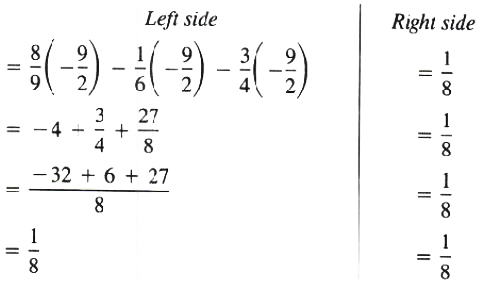The solution set is { 92}.

Let’s see how our Linear equation solver solves this and similar equations. Click on "Solve Similar" button to see more examples.

EXAMPLE List the elements in the set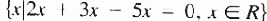Solution  Consider the statement

2 x+3 x-5 x=0

(2+3-5) x=0

0 x=0

Since 0 x=0 is true for any real value of x, we have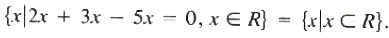EXAMPLE List the elements in the set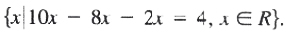Solution  Consider the statement

10 x-8 x-2 x = 4

(10-8-2) x = 4

0 x = 4

Since 0 x=4 is not true for any real value of x, we have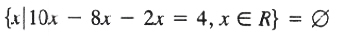Sometimes both sides of the equation contain terms that have the variable as a factor and also terms that do not have the variable as a factor. To ﬁnd the solution set of the equation, form an equivalent equation that has all terms with the variable as a factor on one side of the equation. The terms not having the variable as a factor must appear on the other side.

The equivalent equation can be formed by adding the negative (additive inverse) of the terms to both sides of the equation.

Consider the equation 8 x-5 = 6 x+7

Add (+ 5) to both sides: 8 x-5+5 = 6 x+7+5

8 x+0 = 6 x+12

8 x = 6 x+12

Add ( 6 x) to both sides: 8 x+( 6 x) = 6 x+12+( 6 x)

2 x = 12

x=6

The solution set is {6}.

Remark It is important to realize the difference between the two equations

3 x=15 and 3+x=15

In 3 x=15, the 3 is the coefficient of x; thus to solve for x, multiply both sides of the equation by (13).

13 (3 x)-13 (15)

x=5

The solution set is {5}.

In 3+x=15, the 3 is a term; thus to solve for x, add (-3) to both sides of the equation.

3+x+( 3)=15+( 3)

x=12

The solution set is {12}.

EXAMPLE Solve the equation 2 x-x-3 = 10+7 x-4

Solution  Add (+ 3-7 x) to both sides of equation.

2 x-x-3+(+ 3-7 x)-10+7 x-4+(+ 3-7 x)

2 x-x-3+3-7 x = 10+7 x-4+3-7 x

6 x=9

x = (96) = (32)

The solution set is { 32}.

Note  When the equation contains mixed numbers, change the mixed numbers to improper fractions.

EXAMPLE Solve the equation 312 x 223 x 7 = x6+ 123.

Solution   First change the mixed numbers to improper fractions

72 x-83 x-7 = x6+53

Multiply both sides of the equation by the least common multiple of 2,3,6, and 3, which is 6.

61 (72 x-83 x-7) = 61 (x6+53)

61 (72 x)+61 ( 83 x)+61 ( 7) = 61 (x6)+61 (53)

21 x-16 x-42 = x+10

Add (+ 42-x) to both sides of equation.

21 x-16 x-42+42-x = x+10+42-x

4 x=52

x=13

The solution set is {13}.

Let’s see how our Linear equation calculator solves this and similar equations. Click on "Solve Similar" button to see more examples.

 ← Previous Tutorial Next Tutorial →